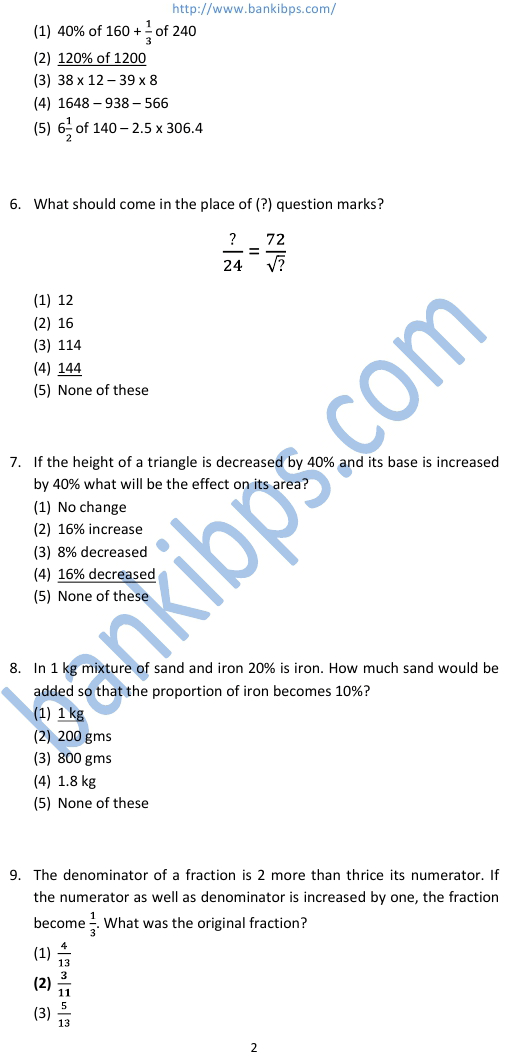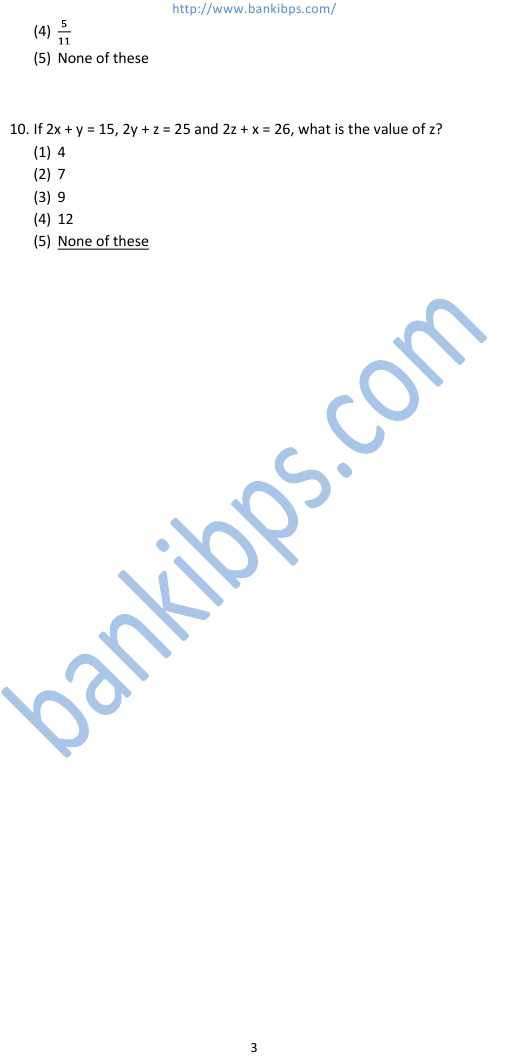# Bank Aptitude Test Questions and Answers

1. The sum of three consecutive even number is 14 less than one-forth of 176. What is the middle number? (1) 8 (2) 10 (3) 6 (4) Data inadequate (5) None of these bank aptitude test questions and answers 2. When 35 percent of a number is added to another number the second number increased by its 20%. What is the ratio between the second number rand the first number? (1) 4:7 (2) 7:4 (3) 8:5 (4) Data inadequate (5) None of these 3. The difference between the digits of a two-digit number is one-ninth of the difference between the original number and the number obtained by interchanging the positions of the digits. What definitely is the sum of the digits of that number? (1) 5 (2) 14 (3) 12 (4) Data inadequate (5) None of these 4. Assuming that A, B and C are different single-digit numerical values other than what is already used in the following equation, what number C definitely cannot be?
Practice Exercise - 326 [ Aptitude ]## bank aptitude test questions and answers

### aptitude tests practice

#### online aptitude practice test

##### aptitude practice tests
###### sample of aptitude test questions for banks
aptitude tests practice. online aptitude practice test. aptitude practice tests. practice tests for gre. bank aptitude test papers. aptitude test question papers with answers. aptitude test papers with answers pdf. gmat aptitude test questions. sample aptitude test questions and answers for banks. sample aptitude test questions for banks. sample of aptitude test questions for banks.